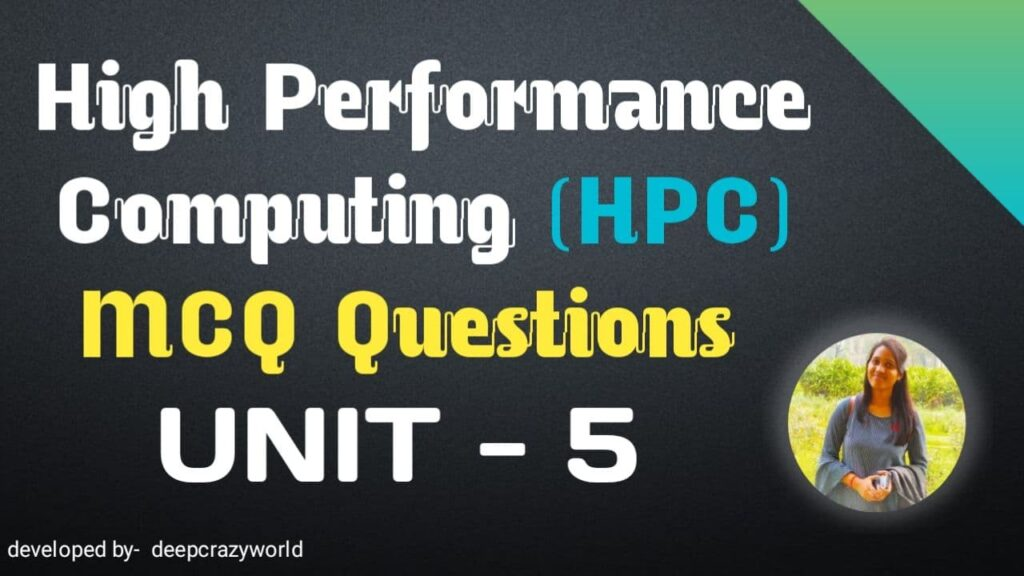# High performance computing MCQ Unit 5 | HPC MCQ

High Performance Computing MCQ Questions and Answers UNIT – 5 What is High performance computing ? HPC – High Performance Computing is the use of parallel processing for running advanced application programs efficiently, reliably and quickly. The term applies especially to systems that function above a teraflop or 1012 floating-point operations per second. The term HPC is occasionally used as a synonym for supercomputing, although technically a supercomputer is a system that performs at or near the currently highest operational rate for computers. Some supercomputers work at more than a petaflop or 1015 floating-point operations per second.

## High Performance Computing MCQ UNIT – 5

high performance computing mcq questions and answers

1. In _, the number of elements to be sorted is small enough to fit into the process’s main memory.

internal sorting
internal searching
external sorting
external searching

1. __ algorithms use auxiliary storage (such as tapes and hard disks) for sorting because the number of elements to be sorted is too large to fit into memory.

internal sorting
internal searching
External sorting
external searching

1. __ can be comparison-based or noncomparison-based.

searching
Sorting
both a and b
none of above

1. The fundamental operation of comparison-based sorting is __

compare-exchange
searching
Sorting
swapping

1. The complexity of bubble sort is Θ(n^2)

TRUE
FALSE

1. Bubble sort is difficult to parallelize since the algorithm has no concurrency.

TRUE
FALSE

1. Quicksort is one of the most common sorting algorithms for sequential computers because of its simplicity, low overhead, and optimal average complexity.

TRUE
FALSE

1. The performance of quicksort depends critically on the quality of the __

non-pivote
pivot
center element
len of array

1. the complexity of quicksort is O(nlog n)

TRUE
FALSE

1. DFS begins by expanding the initial node and generating its successors. In each subsequent step, DFS expands one of the most recently generated nodes.

TRUE
FALSE

## high performance computing mcq sppu

1. The main advantage of __ is that its storage requirement is linear in the depth of the state space being searched.

BFS
DFS
a and b
none of above

1. _ algorithms use a heuristic to guide search.

BFS
DFS
a and b
none of above

1. If the heuristic is admissible, the BFS finds the optimal solution.

TRUE
FALSE

1. The search overhead factor of the parallel system is defined as the ratio of the work done by the parallel formulation to that done by the sequential formulation

TRUE
FALSE

1. The critical issue in parallel depth-first search algorithms is the distribution of the search space among the processors.

TRUE
FALSE

1. Graph search involves a closed list, where the major operation is a _

sorting
searching
lookup
none of above

1. Breadth First Search is equivalent to which of the traversal in the Binary Trees?

Pre-order Traversal
Post-order Traversal
Level-order Traversal
In-order Traversal

1. Time Complexity of Breadth First Search is? (V – number of vertices, E – number of edges)

O(V + E)
O(V)
O(E)
O(V*E)

1. Which of the following is not an application of Breadth First Search?

When the graph is a Binary Tree
When the graph is a Linked List
When the graph is a n-ary Tree
When the graph is a Ternary Tree

high performance computing mcq questions

1. In BFS, how many times a node is visited?

Once
Twice
Equivalent to number of indegree of the node
Thrice

Answer: Equivalent to number of indegree of the node

1. Is Best First Search a searching algorithm used in graphs.

TRUE
FALSE

1. The critical issue in parallel depth-first search algorithms is the distribution of the search space among the processors.

TRUE
FALSE

1. Graph search involves a closed list, where the major operation is a _

sorting
searching
lookup
none of above

1. Which of the following is not a stable sorting algorithm in its typical implementation.

Insertion Sort
Merge Sort
Quick Sort
Bubble Sort

1. Which of the following is not true about comparison based sorting algorithms?

The minimum possible time complexity of a comparison based sorting algorithm is O(nLogn) for a random input array
Any comparison based sorting algorithm can be made stable by using position as a criteria when two elements are compared
Counting Sort is not a comparison based sorting algortihm
Heap Sort is not a comparison based sorting algorithm.

Answer: Heap Sort is not a comparison based sorting algorithm.

Data analytics mcqs with answers for SPPU

Digital Image Processing MCQ | Basics Of Image Sampling & Quantization

Basic of Digital Image Processing

ALL unite digital Image Processing MCQ